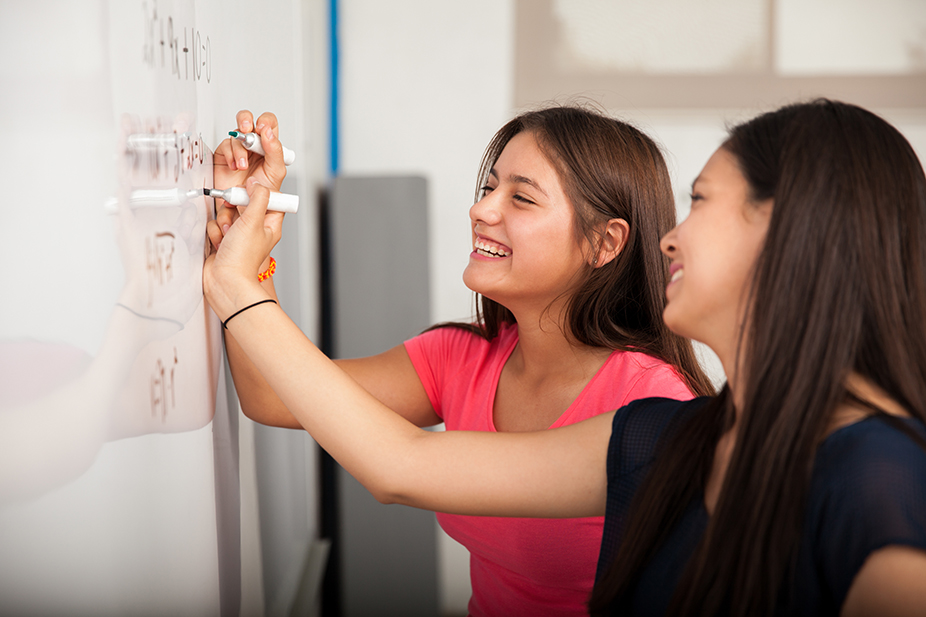# 10 Tips to Improve Math Skills for ScienceMany students enter into scientific study without basic mathematical skills, despite mathematics being an integral part of science. Without an understanding of Logarithms; chemistry students will struggle when learning about buffers. Biology students, using microscopes need to understand how to multiply by 10 for total magnification. Basic algebra is required to solve mass in physics. Many science students begin to panic when they learn that they can’t use their calculators on their exams. To help you improve your students mathematical skills and reduce stress come exam time, we have provided a few tips and tricks to guide your students through fundamental maths skills.

1. Never operate under the assumption that students learned (or retained) maths skills and knowledge from a previous maths classes.
2. To identify knowledge gaps and where your students struggle, conduct a simple math quiz during the first week of the semester. Design the quiz around the basic mathematical concepts imbedded within the subject. No more than 10 questions, focus on single-digit numbers unless specifically testing students on fractions, decimals, or powers of 10.
3. Collaborate with Mathematics teachers to formulate a plan to teach the required maths concepts. Maths teachers are a great resource and can potentially provide manipulatives to demonstrate a concept. Providing sample lab data will help maths teachers aid in writing math questions.
4. Start each topic with an overview of the required math knowledge for the topic. This may include a maths lesson. Ensure students understand logarithms before learning about buffers. Review exponents before tackling PCR. Students will develop a deeper understanding of the scientific concepts if the have comprehension of the maths behind it.
5. Gain students confidence in estimating. Estimation will assist students substantially in test and exam taking. When completing multiple choice questions, students will be able to identify incorrect answers using estimation; making them choosing right answer far more likely. One way you can introduce estimation into your science class is, asking students to predict if a variable will increase or decrease in gas laws before they are given a change to solve the problem methodically.
6. Combine maths and demonstrations to reinforce scientific concepts. Data from demonstrations provides an opportunity to relate to real world mathematical questions.
7. Measurements of central tendency; mean, median, and mode are critical knowledge for students. Whenever the opportunity arises, reinforce their understanding of them and discuss when it is appropriate to use each method.
8. Graphs provide a great intersection of numbers and visual communication; making information to understand. They can illustrate the relationship between mathematics and science. When relevant to the lesson plan, task students with drawing graphs of their data in sample data sets on an XY scatter plot.
9. Using familiar items such as an egg carton with 12 eggs can be used to teach unfamiliar concepts such as the concept of a mole. Find your own methods of using everyday objects to teach more complex concepts.
10. To improve your student’s mathematical competencies toward scientific study, it is absolutely critical to consistently and frequently reinforce their knowledge and skills.